# Force

## Key Stage 2

### Meaning

A force is something which can make an object; speed up, slow down or change shape.

Noun: Force

A force can make an object speed up. When you let go of a pencil it will fall because a force called weight is making it speed up.
A force can make an object slow down. When you roll a ball on the carpet it will slow down and stop because a force called friction has slowed it down.
A force can change the shape of an object. When you tip a spoon of honey the honey will change shape and stretch because a force called weight pulls it into a long shape before dripping.

## Key Stage 3

### Meaning

A force is an influence that can change the speed, direction of motion or shape of an object.

The scientific units of force is the Newton (N). However, force can also be measured in pounds (lb) and ounces (Oz).
Forces can be measured using a Newton Meter.
Forces can be split into two groups, Contact Forces and Non-contact Forces.
 Contact Forces Non-contact Forces Friction Air Resistance Water Resistance Upthrust Driving Force Thrust Lift Gravitational Force (Weight) Magnetic Force Electrostatic Force
Forces can cause an object to accelerate.
A pair of opposing forces can twist, stretch or squash an object. This is known as deformation.

### Force Arrows

Forces can be represented in a diagram with arrows that show the magnitude and direction of the force. A bigger arrow means a bigger force.
 A boat that is not moving. A tennis ball falling. A bowling ball moving to the right.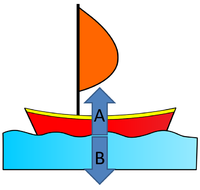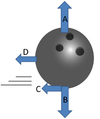A = Upthrust B = Weight A = Air Resistance B = Weight A = Normal Contact Force B = Weight C = Friction D = Air Resistance A submarine travelling to the left. A plane flying to the right. A car driving to the right.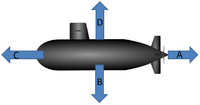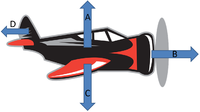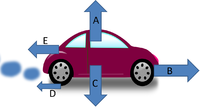A = Water Resistance B = Weight C = Thrust D = Upthrust A = Lift B = Thrust C = Weight D = Air Resistance A = Normal Contact Force B = Driving Force C = Weight D = Friction E = Air Resistance

## Key Stage 4

### Meaning

A force is a vector quantity that can affect the speed, direction of motion or shape of an object.

A force can be measured using a Newton Meter.
The SI Unit of force is the Newton (N).
A force is a vector quantity because it has both magnitude and direction.
Forces follow Newton's Third Law which states that if an object 'A' exerts a force on object 'B'; then object 'B' will exert a force on object 'A' which is equal in magnitude but opposite in direction to the original force. These are sometimes called an action force and a reaction force. This means:
When you push a wall with a force of 5N (Action Force), the wall pushes back with a force of 5N (Reaction Force).
If 'head' a football with a force of 10N then your head provides a force of 10N (Action Force) to the ball and the ball provides a force of 10N to your head (Reaction Force).
Forces can cause an object to accelerate.
A pair of opposing forces applied to different parts of an object can twist, stretch or squash the object. This is known as deformation.

### Forces as Vectors

Forces have both magnitude and direction so they are considered vectors.

#### Positive and Negative Direction

When two forces act in opposite directions, along a line, one direction is chosen to be a positive direction and the other is chosen to be a negative direction, just as on a number line in maths.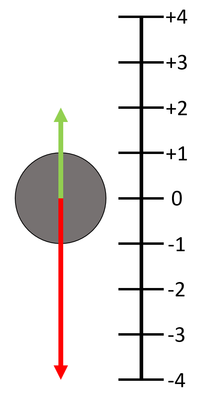If the 'up' direction is chosen to be positive then any vector pointing 'up' has a positive value and any vector pointing 'down' has a negative value. In this diagram the green vector has a value of +2 while the red vector has a value of -4. If the number lines represent the number of Newtons force then the Resultant Force is the two values added together. FResultant = (+2N) + (-4N) FResultant = -2N upwards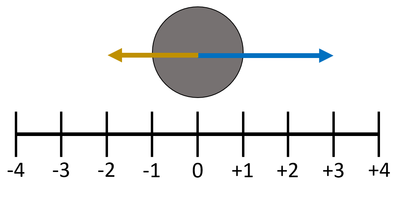If the 'right' direction is chosen to be positive then any vector pointing 'right' has a positive value and any vector pointing 'left' has a negative value. In this diagram the blue vector has a value of +3 while the yellow vector has a value of -2. If the number lines represent the number of Newtons force then the Resultant Force is the two values added together. FResultant = (+3N) + (-2N) FResultant = 1N to the right

#### Forces on a Free Body Diagram

To show force vectors a Free Body Diagram can be used, this indicates the magnitude and direction of the forces.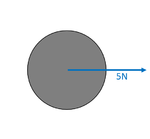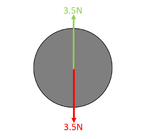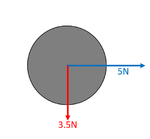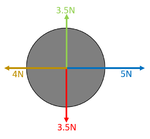This diagram shows an object with a vector force of 5N magnitude directed horizontally to the right. This diagram shows an object with 2 forces of 5N magnitude in opposite directions, vertically. This diagram shows an object with 2 forces. One of 5N magnitude directed horizontally to the right, the other 3.5N directed vertically down. This diagram shows an object with 4 forces with pairs acting in opposing directions. The resultant force here is 1N horizontally to the right.

#### Forces on a Scale Diagram

Forces can be added using a scale diagram.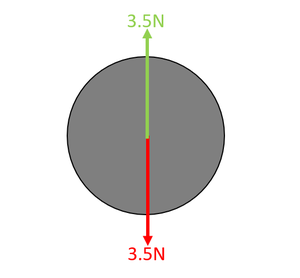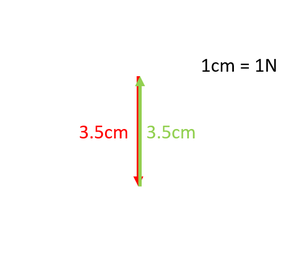The two vector forces drawn to a 'scale' on a Scale Diagram. This scale is 1N = 1cm. The two forces can be added by placing the start of one vector force at the end of the other. This Scale Diagram shows that the two forces cancel one another out so the net force is zero.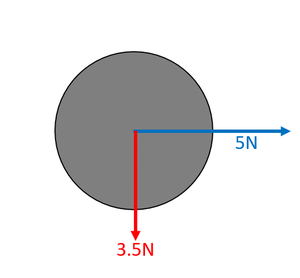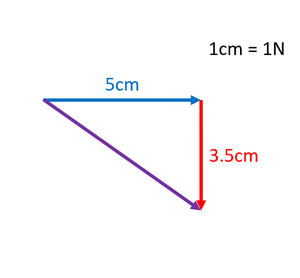The two vector forces drawn to a 'scale' on a Scale Diagram. This scale is 1N = 1cm. The two forces can be added by placing the start of one vector force at the end of the other. This Scale Diagram shows that the two arrows add to make a length of 6.1cm, which represents 6.1N, in a direction of 35° down from the horizontal.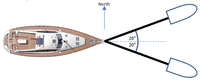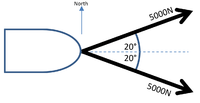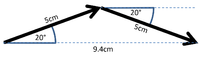A boat is being pulled by two separate tug boats attached by a cable. The tension in each cable is 5000N. The tug boats and cables are replaced by vector forces from the boat. The two forces are redrawn in a a Scale Diagram with scale is 1000N = 1cm placing the start of one vector force at the end of the other. This Scale Diagram shows that the two arrows add to make a length of 9.4cm, which represents 9400N, in a direction of 90° from the North which is an Easterly direction.

#### Resolving Forces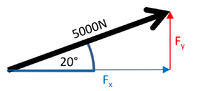A boat is being pulled by two separate tug boats attached by a cable. The tension in each cable is 5000N. The tug boats and cables are replaced by vector forces from the boat. One of the forces is redrawn as the hypotenuse of a right angle triangle with height in the y-axis and base in the x-axis. The total force North can be calculated by finding the height of each triangle. $$F_y=5000\sin20$$ $$F_y=1710N$$ The force from the top tug boat is +1710N to the North and the force from the bottom tug boat is -1710N to the North]] so the total force North is zero. The total force East can be calculated by finding the base of each triangle. $$F_x=5000\cos20$$ $$F_x=4698N$$ The force from the top tug boat is +4698N to the East and the force from the bottom tug boat is +4698N to the East so the total force East is 9396N.